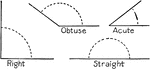### Angles

Four types of angles: right, obtuse, acute, and straight.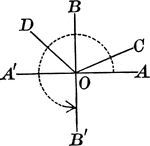### Acute, Obtuse, Straight, Right, And Reflex Angles

An illustration showing acute, obtuse, straight, right, and reflex angles.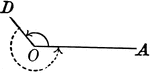### Obtuse And Reflex Angles

An illustration showing an angle the is greater than a right angle and less than a straight angle. This…### Flashcard of an Obtuse angle

A flashcard featuring an illustration of an Obtuse angle### Flashcard of an Obtuse Triangle

A flashcard featuring an illustration of an Obtuse Triangle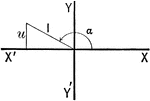Illustration of an angle with the terminal side used to draw a triangle in quadrant II.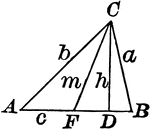### Triangle With Height and Median

Triangle with height/altitude h and median m.### Triangle With Dimensions

Triangle with dimensions labeled. Triangle can be used to calculate area.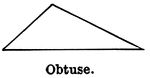### Obtuse Triangle

Illustration showing an obtuse triangle (one that has one obtuse angle).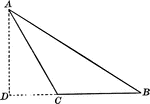### Obtuse Triangle

Obtuse triangle with altitude drawn.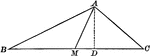### Obtuse Triangle

Obtuse triangle with altitude and one median drawn.### Obtuse Triangle With Height Drawn

Triangle with sides a,b,c and height/altitude h.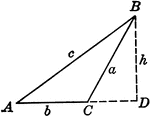### Obtuse Triangle ABC With Height h

Triangle ABC with sides a,b,c labeled and angles A,B,C labeled and h labeled as height.